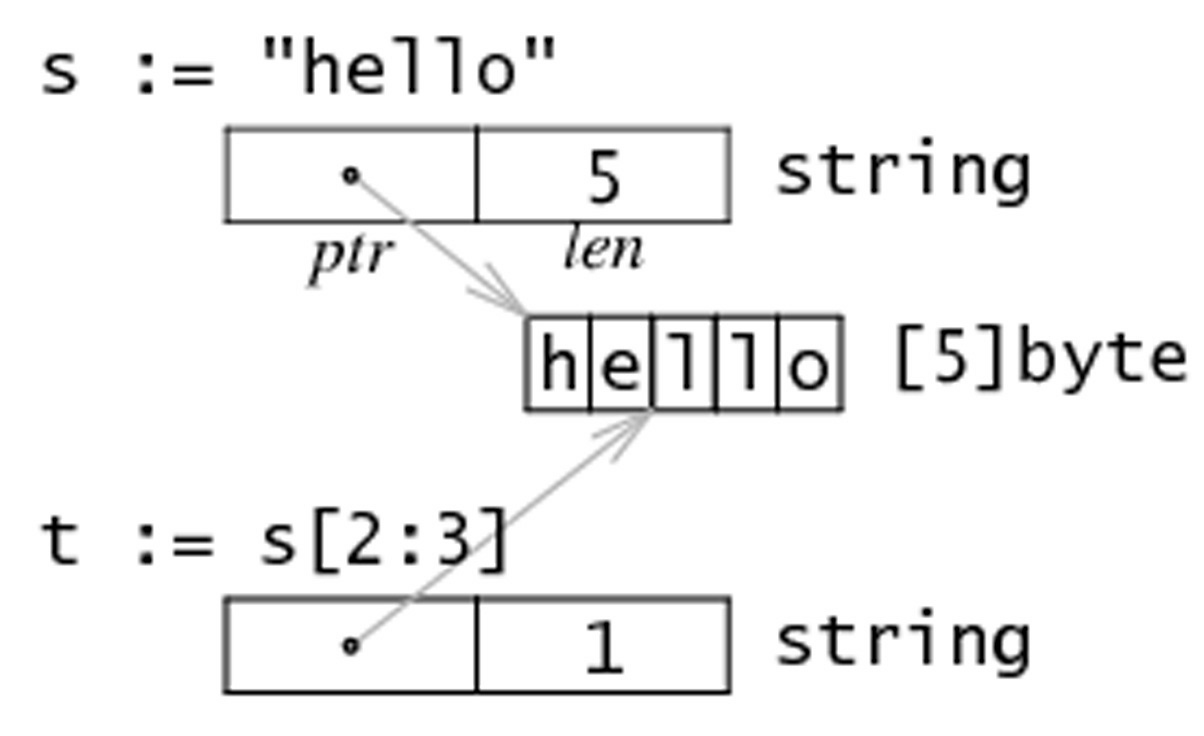# 躲雨的屋檐

## Golang入门笔记-05-数组和切片### 数组

#### 初始化

``````var variable [len]type
``````

``````var arr1 int
``````

``````var numArray = [...]int{1, 2, 3}
``````

``````a := [...]string{0: "北京", 1: "上海"} // 索引 0 对应的元素为"北京"，1 对应的元素为"上海"
``````

#### 遍历数组

• 普通 for 循环

``````package main

import "fmt"

func main() {
a := [...]int{2, 4, 6, 8, 10}

for i := 0; i < len(a); i++ {
fmt.Println(a[i])
}
}
``````
• for-range 循环

``````package main

import "fmt"

func main() {
a := [...]int{2, 4, 6, 8, 10}

for k, v := range a {
fmt.Println(k, v)
}
}
``````

### 切片

#### 概念

`0 <= len(s) <= cap(s)`

``````var variable []type
``````

``````var s = []int{1, 2, 3}
``````

`arr` 是数组，可以通过切割数组来声明切片 `s`，如：

``````var arr = [...]int{1, 2, 3, 4, 5}
var s []int = arr[0:3] // 声明切片 s，s 由 arr 中索引 0 到 2 的元素构成
var s1 []int = arr[:]  // s1 由 arr 所有元素构成
var s2 []int = arr[2:] // s2 由 arr 中索引 2 开始到最后的元素构成
var s3 []int = arr[:3] // s3 由 arr 中索引 0 ~ 2 的元素构成
``````

#### 用 make() 创建切片

``````var s []type = make([]type, len)
``````

``````package main

import "fmt"

func main() {
var slice1 []int = make([]int, 10)

for i := 0; i < len(slice1); i++ {
slice1[i] = 5 * i
}

for i := 0; i < len(slice1); i++ {
fmt.Printf("Slice at %d is %d\n", i, slice1[i])
}
fmt.Printf("\nThe length of slice1 is %d\n", len(slice1))
fmt.Printf("The capacity of slice1 is %d\n", cap(slice1))
}
``````

``````Slice at 0 is 0
Slice at 1 is 5
Slice at 2 is 10
Slice at 3 is 15
Slice at 4 is 20
Slice at 5 is 25
Slice at 6 is 30
Slice at 7 is 35
Slice at 8 is 40
Slice at 9 is 45
The length of slice1 is 10
The capacity of slice1 is 10
``````

``````slice1 := make([]type, length, cap) // type 为类型，length 为初始长度, cap 为切片容量
``````

#### 切片重组

``````sl = sl[0 : len(sl)+1]
``````

``````package main

import "fmt"

func main() {
slice1 := make([]int, 0, 10)
// load the slice, cap(slice1) is 10:
for i := 0; i < cap(slice1); i++ {
slice1 = slice1[0 : i+1]
slice1[i] = i
fmt.Printf("The length of slice is %d\n", len(slice1))
}

// print the slice:
for i := 0; i < len(slice1); i++ {
fmt.Printf("Slice at %d is %d\n", i, slice1[i])
}
}
``````

``````The length of slice is 1
The length of slice is 2
The length of slice is 3
The length of slice is 4
The length of slice is 5
The length of slice is 6
The length of slice is 7
The length of slice is 8
The length of slice is 9
The length of slice is 10
Slice at 0 is 0
Slice at 1 is 1
Slice at 2 is 2
Slice at 3 is 3
Slice at 4 is 4
Slice at 5 is 5
Slice at 6 is 6
Slice at 7 is 7
Slice at 8 is 8
Slice at 9 is 9
``````

#### 切片的复制与追加

``````package main

import "fmt"

func main() {
sl_from := []int{1, 2, 3}
sl_to := make([]int, 10)

n := copy(sl_to, sl_from)
fmt.Println(sl_to)
fmt.Printf("Copied %d elements\n", n) // n == 3

sl3 := []int{1, 2, 3}
sl3 = append(sl3, 4, 5, 6)
fmt.Println(sl3) // 1, 2, 3, 4, 5, 6
}
``````

`append` 函数可以将多个相同类型的元素追加到切片后面，同时返回新的切片。若切片的容量不够，`append` 会分配新的切片保证能存储原切片和新元素。

``````x = append(x, y...)
``````

``````func AppendByte(slice []byte, data ...byte) []byte {
m := len(slice)     // 原切片长度
n := m + len(data)  // 新切片长度
if n > cap(slice) { // 若新切片长度大于切片容量，手动扩容
newSlice := make([]byte, (n+1)*2)
copy(newSlice, slice)
slice = newSlice
}
// 复制元素
slice = slice[0:n]
copy(slice[m:n], data)
return slice
}
``````

### 字符串、数组和切片的应用

#### 从字符串生成字节切片

`s` 为一个字符串，可以通过 `c := []bytes(s)` 来获取 `s` 对应的字节切片。也可以通过 `copy` 函数来实现：`copy (b []byte, s string)`

#### 截取字符串

`substr := str[start:end]` 可以从字符串 `str ` 获取到从索引 `start ` 开始到 `end-1` 位置的子串；

`str[start:]` 则表示获取从 `start` 开始到 `len(str)-1` 位置的子串；

`str[:end]` 表示获取从 0 开始到 `end-1` 的子串。

#### 字符串和切片的内存结构#### 修改字符串的字符

Go 语言的字符串是不可变的，对于 `str[index]`，我们不能执行这样的语句：

``````str[index] = 'A'
``````

``````s := "hello"
c := []byte(s)
c = 'm'
s2 := string(c) // "mello"
``````

#### append 函数

1. 切片 `b` 的元素追加到切片 `a` 之后：`a = append(a, b...)`

2. 复制切片 `a` 的元素到新的切片 `b` 上：

``````b = make([]T, len(a))
copy(b, a)
``````
3. 删除位于索引 `i` 的元素：`a = append(a[:i], a[i+1:]...)`

4. 切除切片 `a` 中从索引 `i``j` 位置的元素：`a = append(a[:i], a[j:]...)`

5. 为切片 `a` 扩展 ` j` 个元素长度：`a = append(a, make([]T, j)...)`

6. 在索引 `i` 的位置插入元素 `x``a = append(a[:i], append([]T{x}, a[i:]...)...)`

7. 在索引 `i` 的位置插入长度为 `j` 的新切片：`a = append(a[:i], append(make([]T, j), a[i:]...)...)`

8. 在索引 `i` 的位置插入切片 `b` 的所有元素：`a = append(a[:i], append(b, a[i:]...)...)`

9. 取出位于切片 `a` 最末尾的元素 `x``x, a = a[len(a)-1], a[:len(a)-1]`

10. 将元素 `x` 追加到切片 `a``a = append(a, x)`

#### 切片和垃圾回收

``````var digitRegexp = regexp.MustCompile("[0-9]+")

func FindDigits(filename string) []byte {
return digitRegexp.Find(b)
}
``````

``````func FindDigits(filename string) []byte {